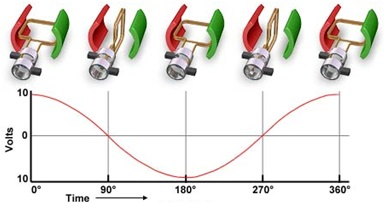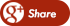﻿ Generation of a Sine Wave of VoltageBack

## Generation of a Sine Wave of VoltageGeneration of a Sine Wave of Voltage

There are two facts that the voltage developed in a coil of a generator changes; the first one is it changes in magnitude from instant to instant as varying values of flux are cut per second and the other one is it changes in direction as coil side change positions under north and south poles, implies that alternating emf is generated. This means that the voltage is maximum as mentioned in our last topic here when the position of the coil is and will diminish to zero as the coil rotates clockwise toward the position

Then, as the coil continues to rotate clockwise, the polarities will change. Assuming uniform flux distribution between north and south poles, the generated voltage in a coil located from the vertical will be:e = Em sin α

Consider the figure below for us to analyze why this relationship mentioned above happened.

It was come up to the relationship between instantaneous voltage e and maximum voltage Em is that a coil side such as a, moving tangentially to a circle as indicated, cut lines of force in proportion to its vertical component of the motion. If the vector length ay in the figure above represents a constant rotating velocity, it should be obvious that vector xy is, its vertical component; the vector lengthax is the horizontal component and it emphasize that motion in this direction involves no flux- cutting action. Since the velocity ratio xy/ay=sinα is also a measure of the voltage in coil side a with respect to the maximum voltage (when the coil is located horizontally) it follows that sinα is a varying proportionality factor that equates e to Em.The equation above may be used to determine a succession of generated voltage values in a coil as it rotates through a complete revolution. This is just by computing with its selected angular displacements.

A more convenient way of representing the instantaneous voltage equation mentioned above is to draw a graph to illustrate a smooth variation of voltage with respect to the angular position of the coil, this graph is called a sine wave. The wave repeats itself and it is called a periodic, then each complete succession of values is called a cycle, while each positive or negative half of the cycle is calledalternation.

Now, we can say that an alternating voltage as an emf that varies in magnitude and direction periodically. Then, when the emfs are proportional to the trigonometric sine function, it is referred to a sinusoidal alternating voltage. However, there are also some periodic waves which do not follow this shape and they are called non sinusoidal waves. This topic will be covered when we reached more complicated analysis is AC Circuits.Lets have a practical example of a problem using the equation above just for you to appreciate the presented  formula above:

Problem : The voltage in an ac circuit varies harmonically with time with a maximum of 170V. What is the instantaneous voltage when it has reached 45 degree in its cycle?

Using, e = Em sin α = 170V x sin (45 degree) = 170V x 0.71 = 120 V.In the common 60 cycle ac circuit, there are 60 complete cycle each second; i.e. the time interval of 1 cycle is 1/60 sec. It should be noted that this corresponds to a reversal in a direction of the current every 1/120 sec. (since the direction reverses twice during each cycle).

FOR MORE DETAILS: CLICK HERE...